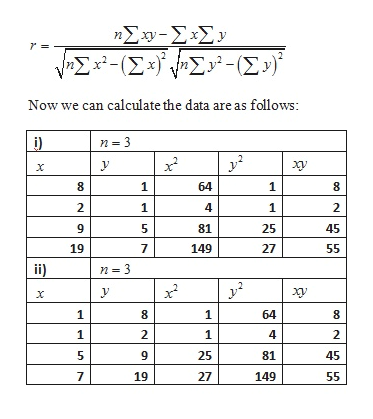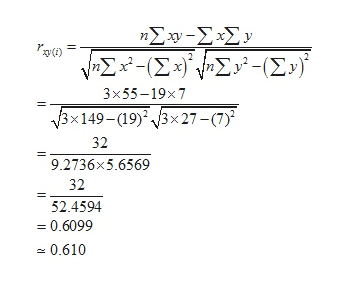# Compute the sample correlation coefficient r for each of the following data sets and show that r is the same for both. (Use 3 decimal places.)(i)x829 y115(ii)x115 y829 r(i) (ii)

Question
9 views
Compute the sample correlation coefficient r for each of the following data sets and show that r is the same for both. (Use 3 decimal places.)
 (i) x 8 2 9 y 1 1 5

 (ii) x 1 1 5 y 8 2 9
 r (i) (ii)
check_circle

Step 1

We know the sample correlation coefficient r between x and y variable as:help_outlineImage TranscriptioncloseηΣ-ΣΧΣy wΣ-- (Σ-)Σ'-Σy Now we can calculate the data are as follows: 2 y X 8 1 64 2 1 4 1 2 5 81 25 45 19 7 149 27 55 ii) n = 3 2 y X 1 8 64 8 1 2 1 2 5 25 81 45 19 27 149 55 LO LC fullscreen
Step 2

Therefore the sample correlation coefficient r between x and y for sample 1:help_outlineImage TranscriptioncloseΣw-ΣΣ νΣ-Σ3Σ- (Σy? Το ) 3x55-19x7 βx 149-(19)*3x27- (7)> 32 9.2736x5.6569 32 52.4594 0.6099 0.610 fullscreen
Step 3

Therefore the sample correlation coefficient ...

### Want to see the full answer?

See Solution

#### Want to see this answer and more?

Solutions are written by subject experts who are available 24/7. Questions are typically answered within 1 hour.*

See Solution
*Response times may vary by subject and question.
Tagged in

### Data